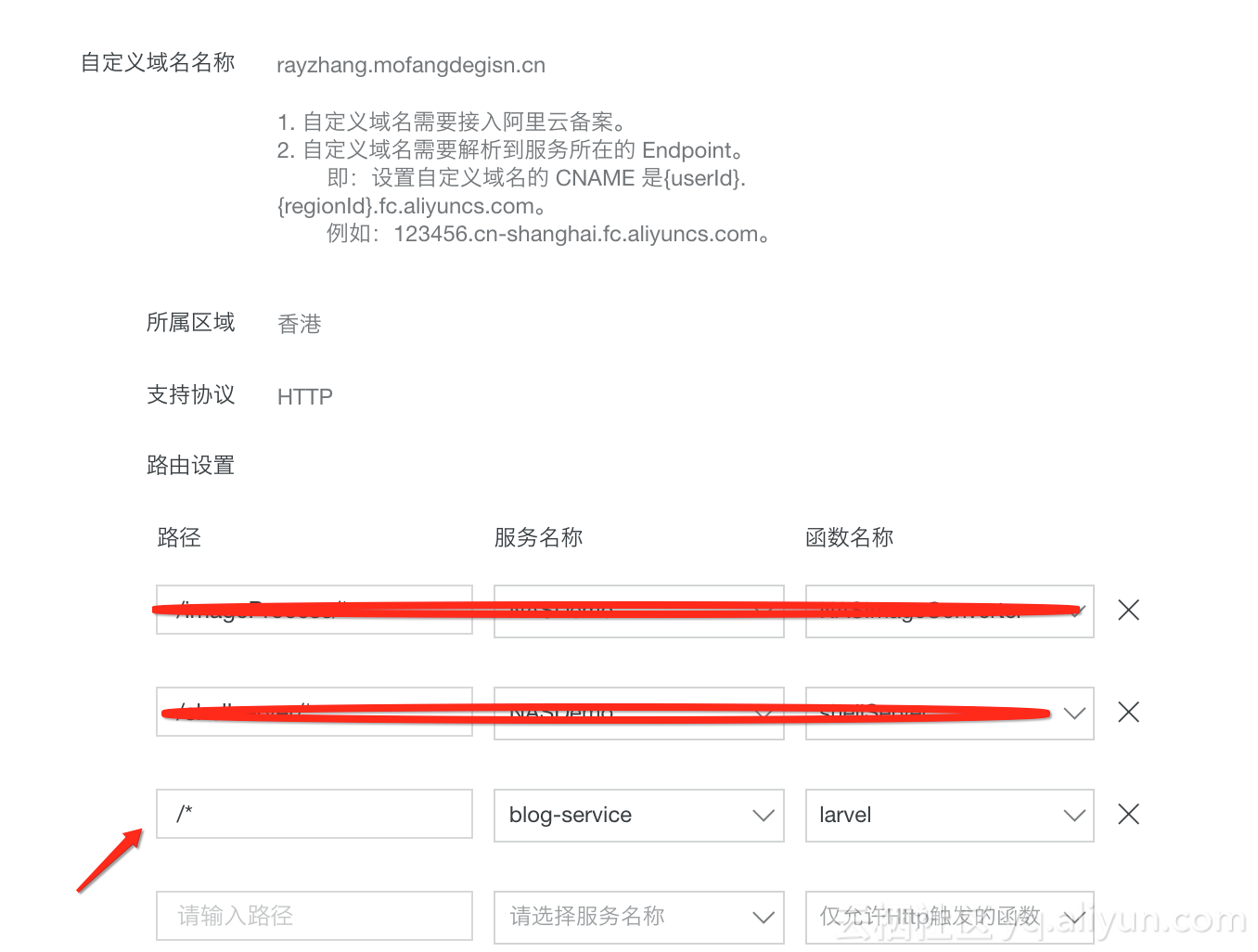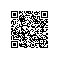# 十分钟上线-函数计算&Laravel的那些事儿

@Deprecated

## FC 运行larvel 流程

• 下载 Learn-Laravel-5，准备好mysql数据库。

• 修改.env， 主要是修改数据库连接相关内容
DB_CONNECTION=mysql
DB_HOST=rm-xxyyyy12583ck110swao.mysql.rds.aliyuncs.com
DB_PORT=3306
DB_DATABASE=fc-larvel
DB_PASSWORD=pwd123456
• php artisan migrate，做数据库初始化工作
• chmod -R 777 storagechmod -R 777 bootstrap/cache, 保证这两个目录可写
• 执行命令php artisan key:generate，生成app_key
• 将 Learn-Laravel-5 工程移到上述配置的 NAS 中, www 表示 Learn-Laravel-5 的工程的根目录。
|-- index.py
|-- www

index.py代码：

# -*- coding: utf-8 -*-
import logging
import os

file = "/mnt/www/"

def mkdir(path):
folder = os.path.exists(path)
if not folder:
os.makedirs(path)

def lsDir():
os.system("ls -ll /mnt/www/")

def handler(event, context):
mkdir(file)
os.system("cp -r /code/www/* /mnt/www/")
os.system("cp -a /code/www/.env /mnt/www/.env")
print(lsDir())
return 'ok'

• 编写函数，代码如下：
laravel 是单入口框架，所有的url都是经过 public/index.php

<?php
use RingCentral\Psr7\Response;

function startsWith($haystack,$needle) {
$length = strlen($needle);
return (substr($haystack, 0,$length) === $needle); } function handler($request, $context): Response{$uri    = $request->getAttribute("requestURI");$isPhpScript = false;
$root_dir = '/mnt/www';$filename = $root_dir . explode("?",$uri);
$filename = rawurldecode($filename);

$pathinfo = pathinfo($filename);
if(!isset($pathinfo['extension'])){$isPhpScript = true;
}else{
$extension = strtolower($pathinfo['extension']);
if($extension == 'php'){$isPhpScript = true;
}
}

$proxy =$GLOBALS['fcPhpCgiProxy'];
//php script
if ($isPhpScript) {$host   = "rayzhang.mofangdegisn.cn";
$resp =$proxy->requestPhpCgi($request,$root_dir, "index.php",
['SERVER_NAME' => $host, 'SERVER_PORT' => '80', 'HTTP_HOST' =>$host,
'SCRIPT_FILENAME' => $root_dir . "/public/index.php", 'SCRIPT_NAME' => "/index.php"], ['debug_show_cgi_params' => true, 'readWriteTimeout' => 20000] ); return$resp;
} else {
// static files, js, css, jpg ...
$handle = fopen($filename, "r");
$contents = fread($handle, filesize($filename)); fclose($handle);
$headers = [ 'Content-Type' =>$proxy->getMimeType($filename), 'Cache-Control' => "max-age=8640000", 'Accept-Ranges' => 'bytes', ]; return new Response(200,$headers, \$contents);
}
}
• 给函数入口配置自定义域名(操作过程请参考：绑定自定义域名示例), 具体配置假设如下：## 总结

FC 可以做为 Web Backend，只需要编写一个函数实现传统 Web 服务器中的 conf 中的逻辑，就可以将一个完整的 Web 工程迁移到 FC ，从而从传统的 Web 网站运维，监控等繁琐的事务中解放出来。使用钉钉扫一扫加入圈子
+ 订阅

Serverless微服务应用实践分享